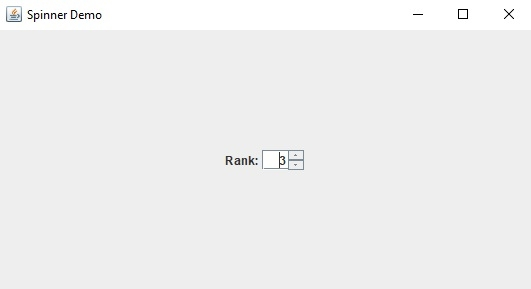# How to limit the values in a Number JSpinner Component with Java?

To limit the values in a number JSpinner component, use the SpinnerNumberModel that allows to set the min, max, step size and even the initial value −

value − current value of the model
min − first number in the sequence
max − last number in the sequence
stepSize − difference between elements of the sequence

Let us set the above values −

int min = 0;
int max = 10;
int step = 1;
int i = 1;
SpinnerModel value = new SpinnerNumberModel(i, min, max, step);

Now, we will set these values to our JSpinner −

JSpinner spinner = new JSpinner(value);

The following is an example to limit the values in a number JSpinner component −

## Example

package my;
import java.awt.GridBagLayout;
import javax.swing.*;
public class SwingDemo {
public static void main(String[] args) {
JFrame frame = new JFrame("Spinner Demo");
JPanel panel = new JPanel();
JLabel label = new JLabel("Rank − ");
panel.setLayout(new GridBagLayout());
int min = 0;
int max = 10;
int step = 1;
int i = 1;
SpinnerModel value = new SpinnerNumberModel(i, min, max, step);
JSpinner spinner = new JSpinner(value);
spinner.setBounds(50, 80, 70, 100);
}